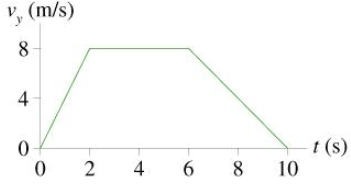# Problem: The figure shows the velocity graph of a 75 kg passenger in an elevator.1) What is the passenger's apparent weight at t = 1.0 s?2) What is the passenger's apparent weight at t = 5.0s?3) What is the passenger's apparent weight at t = 9.0s?

###### FREE Expert Solution

Acceleration:

$\overline{){\mathbf{a}}{\mathbf{=}}\frac{\mathbf{∆}\mathbf{v}}{\mathbf{∆}\mathbf{t}}}$

The apparent weight is the same as the normal force.

95% (302 ratings)###### Problem Details

The figure shows the velocity graph of a 75 kg passenger in an elevator.1) What is the passenger's apparent weight at t = 1.0 s?

2) What is the passenger's apparent weight at t = 5.0s?

3) What is the passenger's apparent weight at t = 9.0s?